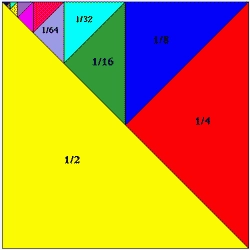# The sum 27

The sum of a geometric progression's second and third terms is six times the fourth term. Find the two possible values of the common ratio.

r1 =  0.5
r2 =  -0.3333

### Step-by-step explanation:Did you find an error or inaccuracy? Feel free to write us. Thank you!

Tips for related online calculators
Are you looking for help with calculating roots of a quadratic equation?
Do you have a linear equation or system of equations and looking for its solution? Or do you have a quadratic equation?